# mohamed82008 / VTKDataIO.jl

A package for reading, writing and visualizing mesh data, and interfacing with Python's VTK.
Julia
Latest commit a93a158 Sep 8, 2017
Type Name Latest commit message Commit time
Failed to load latest commit information.srctest Jul 9, 2017.codecov.yml Jul 6, 2017.gitignore Jul 6, 2017.travis.yml Jul 6, 2017LICENSE.mdREADME.mdREQUIREappveyor.yml Jul 6, 2017

# VTKDataIO

## Overview

VTKDataIO.jl presents a number of input, output and visualization functionalities for geometric meshes with point and cell scalar and vector data. This module attempts to bridge between VTKDataTypes.jl, Julia's native module for representing VTK data types and the Visualization Toolkit (VTK) hence giving access to many of VTK's capabilities to Julia users. VTKDataIO.jl only supports Julia v0.6.

## Summary of capabilities

### Input and output

You can use VTKDataIO.jl to read/write any of the following file formats into/from the corresponding type in VTKDataTypes.jl: vtk, vtu, vtp, vts, vtr, vti, vtm, pvd, stl, ply. You can do this using `read_vtk`, `write_vtk`, `read_stl`, `write_stl`, `read_ply`, and `write_ply`.

### Visualization and rendered output

You can use VTKDataIO.jl to visualize a scalar or vector field with a 3D heat map and a legend. This includes point-based coloring or cell based coloring, as well as other features such as wireframe and glyph representations. The resulting visualization can be written to ply or x3d formats. You can do this using `visualize`, `write_x3d`, and `write_ply`. `visualize_3ds` can also be used to visualize 3ds files directly.

### Interfacing with Python's VTK

You can use `PyVTK` to change a Julia native VTK object to a VTK data PyObject that can be used in your own VTK pipeline through PyCall. You can also use `_VTKDataTypes` to change a VTK data PyObject back to Julia's native VTK types.

## Setup

The following are the setup steps in Windows. If you are using other OS and you can't set it up, please let me know and I will try to help if I can. Basically if you can reach step 7, you are ready to use VTKDataIO.jl even if you didn't eactly follow the previous steps!

1. Get VTK on your computer. I tested it using VTK 7.1.1.
2. Get Python 2.7, and add it to your PATH variable (2nd part is optional but recommended)
3. Get Numpy
4. Add the equivalent path to "C:\Program Files\VTK 7.1.1\bin\Lib\site-packages\vtk" to your PYTHONPATH User environment variable on your computer if you are using Windows, or the equivalent in any other OS. Basically make sure when you open a Python shell, and write `import vtk` that it works.
5. Get PyCall.jl
6. Find your computer's python.exe file, e.g. "C:\Python27\python.exe", and run the following 2 commands in Julia replacing the path below with your own and "\" with "\\":
``````ENV["PYTHON"] = "C:\\Python27\\python.exe"
Pkg.build("PyCall")
``````
1. Test the following Julia code and make sure it works.
``````using PyCall
@pyimport vtk as vtk
@pyimport numpy as np
``````

## Test cases

If you run the following code, you should be able to get a cubic mesh with randomly coloured cells.

``````using VTKDataTypes
using VTKDataIO

x = y = z = [-2, -1, 0, 1, 2];
rect = VTKRectilinearData([x,y,z]);
rect.cell_data["Cell scalar"] = reshape([rand() for i in 1:num_of_cells(rect)], cell_extents(rect));
visualize(rect, color="Cell scalar")
``````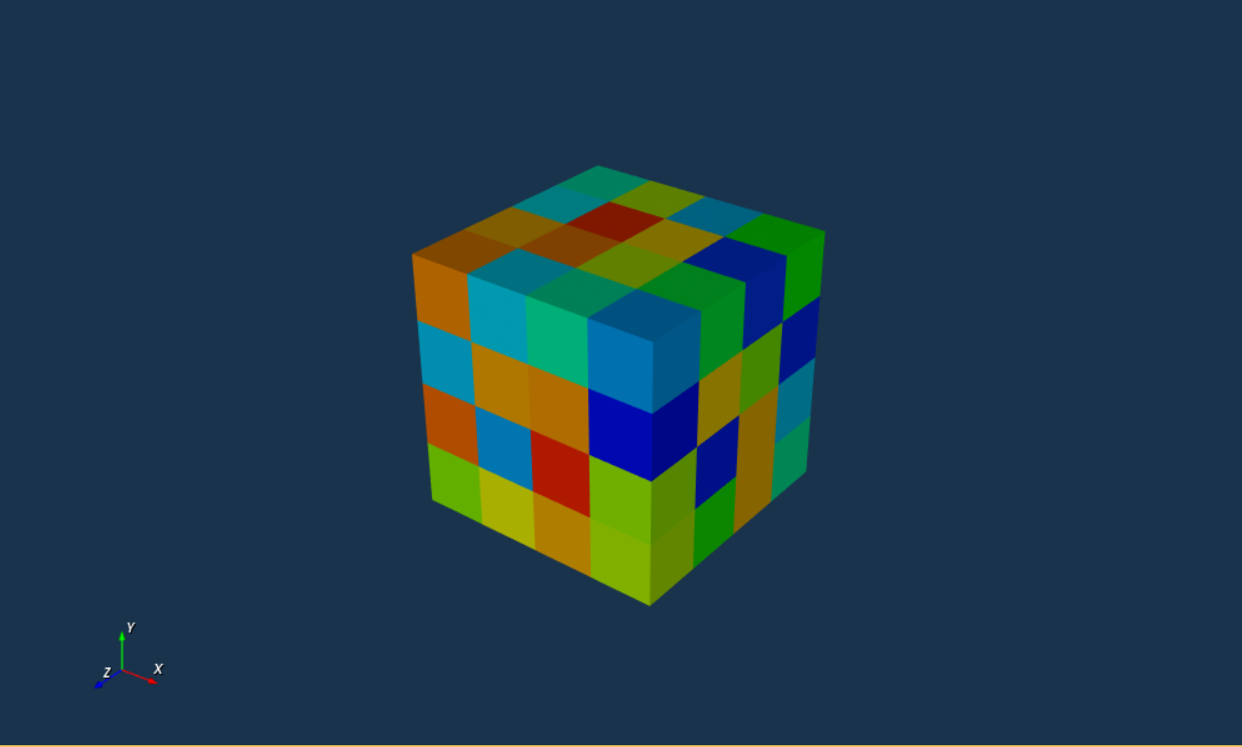You can also do point-based coloring as so:

``````rect.point_data["Point scalar"] = reshape([rand() for i in 1:num_of_points(rect)], extents(rect));
visualize(rect, color="Point scalar")
``````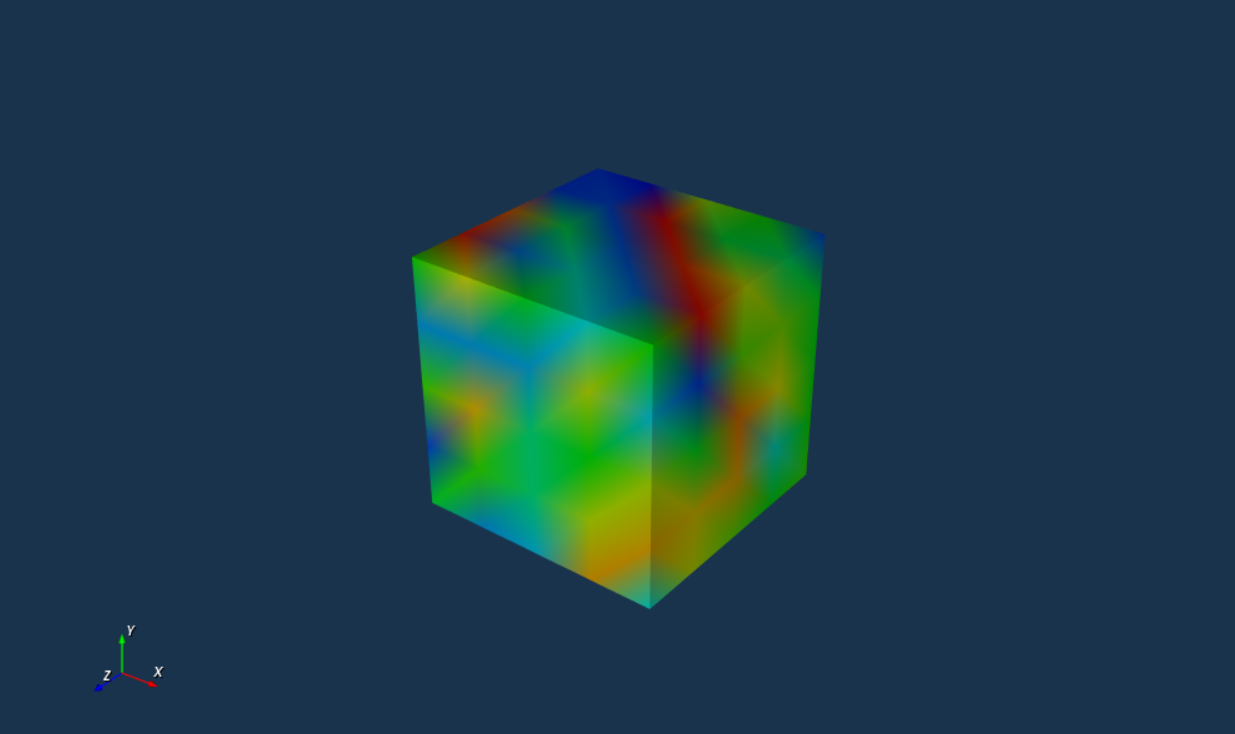Point based vector data can be represented by arrows using the `representation` option.

``````rect.point_data["Point vector"] = reshape([2*rand()-1 for i in 1:3*num_of_points(rect)], (3, extents(rect)...));
visualize(rect, color="Point vector", representation=:glyph, scale_factor=0.5)
``````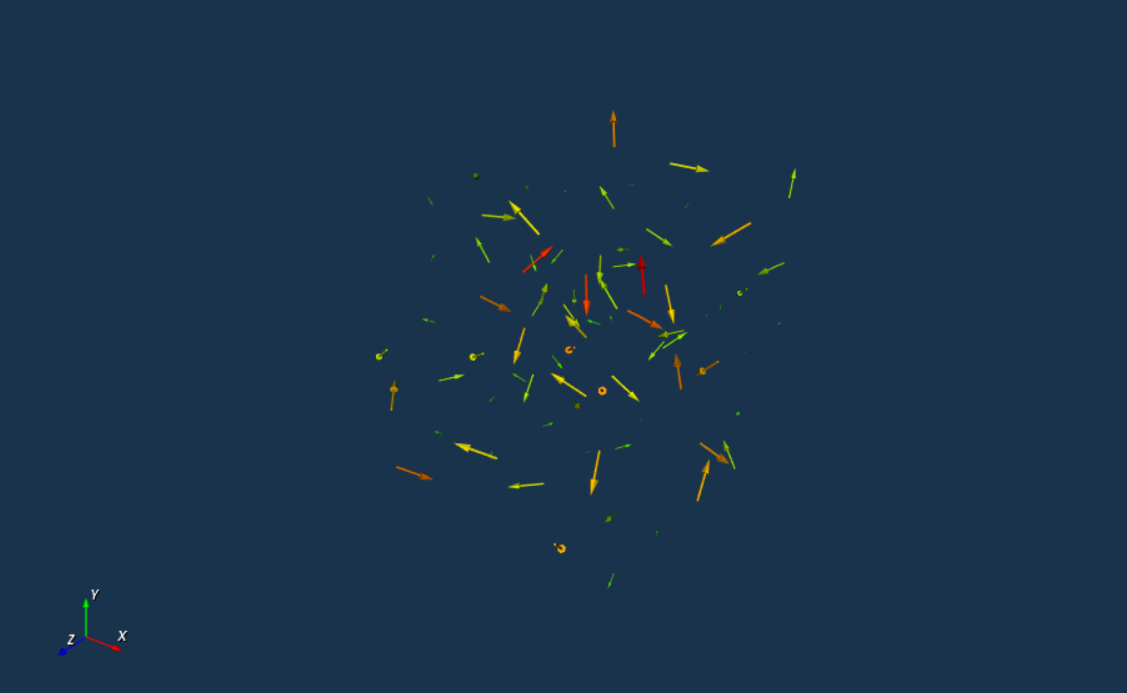Wireframe representation can be used as follows:

``````visualize(rect, color="Point scalar", representation=:wireframe)
``````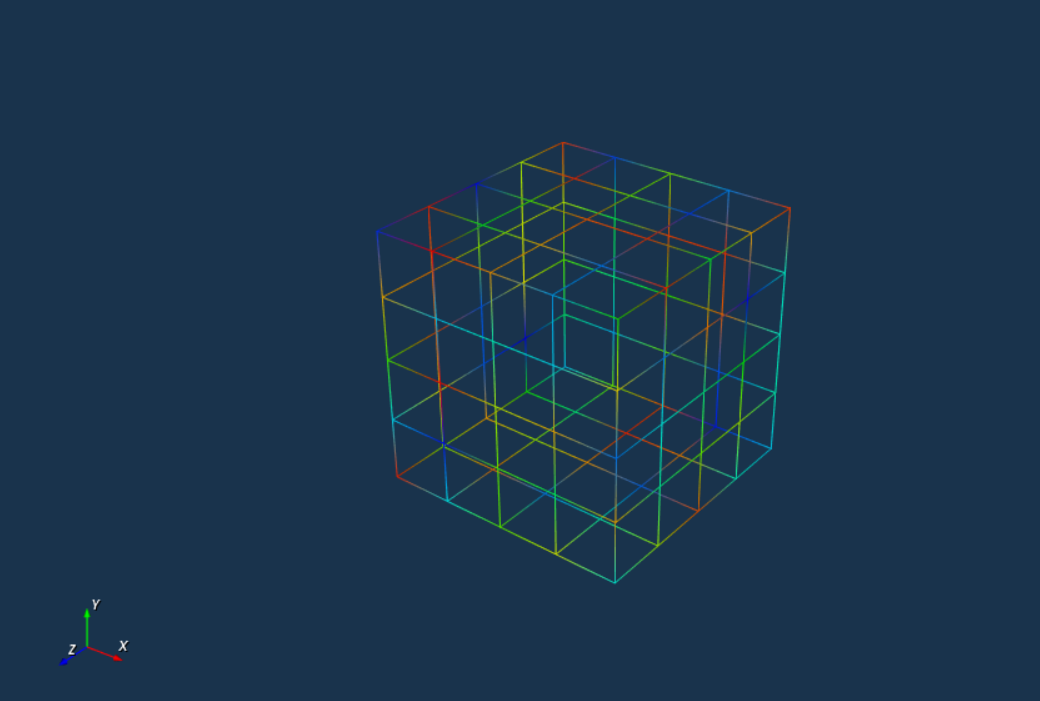If you have the RGB colors data directly, you can inform the `visualize` function to use it using the `RGB = true` option as so:

``````rect.point_data["RGB colors"] = reshape([round(255*rand()) for i in 1:3*num_of_points(rect)], (3, extents(rect)...));
visualize(rect, color="RGB colors", RGB=true)
``````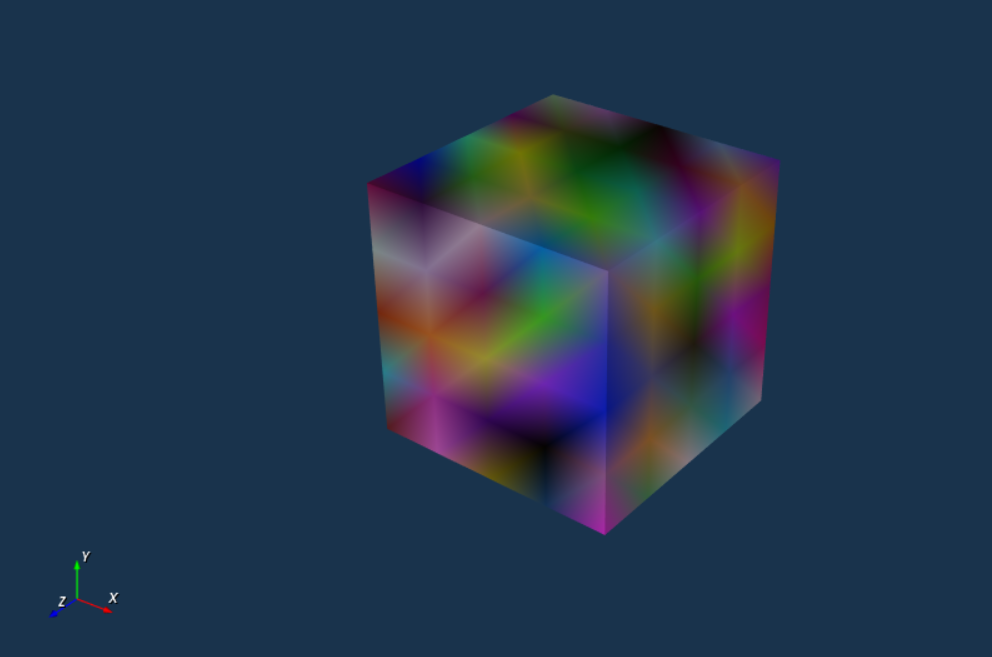You can’t perform that action at this time.